Courses

# Mathematics Test 4 - Matrices And Determinants, Probability, Indefinite Integration

## 30 Questions MCQ Test JEE Main Mock Test Series 2020 & Previous Year Papers | Mathematics Test 4 - Matrices And Determinants, Probability, Indefinite Integration

Description
This mock test of Mathematics Test 4 - Matrices And Determinants, Probability, Indefinite Integration for JEE helps you for every JEE entrance exam. This contains 30 Multiple Choice Questions for JEE Mathematics Test 4 - Matrices And Determinants, Probability, Indefinite Integration (mcq) to study with solutions a complete question bank. The solved questions answers in this Mathematics Test 4 - Matrices And Determinants, Probability, Indefinite Integration quiz give you a good mix of easy questions and tough questions. JEE students definitely take this Mathematics Test 4 - Matrices And Determinants, Probability, Indefinite Integration exercise for a better result in the exam. You can find other Mathematics Test 4 - Matrices And Determinants, Probability, Indefinite Integration extra questions, long questions & short questions for JEE on EduRev as well by searching above.
QUESTION: 1

### If  n ≠ 3k and 1 ,ω,ω2 are the cube roots of unity then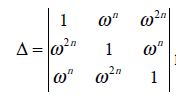has the value

Solution:

Del=(1-w^3n)+w^n(0)+w^2n(w^4n-w^n) =1-(w^3)^n+(w^6)^n-(w^3)^n =1-1+1-1 =0

QUESTION: 2

Solution:
QUESTION: 3

###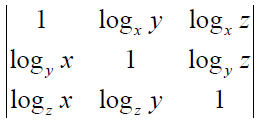x,y,z being +ive equals

Solution:
QUESTION: 4
The roots of the equation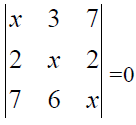are

Solution:
QUESTION: 5
If the three equations are consistent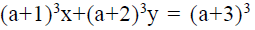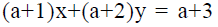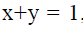, then a =
Solution:
QUESTION: 6

The number of values of k for which the system of equations ( k+1)x + 8y = 4k, kx + (k+3)y = 3k - 1 has inifinitely many solutions is

Solution:

For infinitely many solutions the two equation become identical.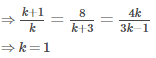QUESTION: 7
If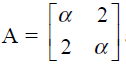and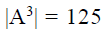, then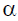is
Solution:
QUESTION: 8

Let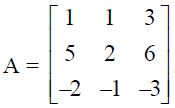. Then A is

Solution:
QUESTION: 9

IF B is a non-singular matrix and A is a square matrix, then  det (B-1 AB)

Solution:
QUESTION: 10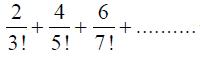will be equal to

Solution:
QUESTION: 11
The sum of the series is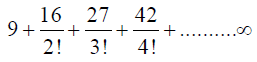Solution:
QUESTION: 12
The sum of the series is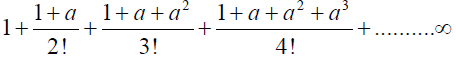Solution:
QUESTION: 13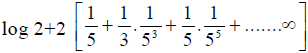is equal to
Solution:
QUESTION: 14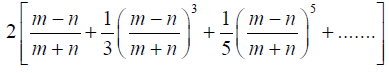is equal to
Solution:
QUESTION: 15

Two cards are drawn at random and without replacement from a pack of 52 playing cards. Find the probability that both the cards are black.

Solution:
QUESTION: 16

Seven chits are numbered 1 to 7. Three are drawn one by one with replacements. The probability that the least number on any selected chit is 5, is

Solution:
QUESTION: 17

A student appear for tests I,II and III. The student is successful if he passes either in tests I and II or tests I and III. The probabilities of the student passing in tests I,II and III are p,q and 1/2 respectively. If the probability that the student is successful is 1/2, then

Solution:
QUESTION: 18

Words from the letters of the word PROBABILITY are formed by talking all at a time.The probability that both B’s are together & both I’s are together is

Solution:
QUESTION: 19

The probability that a person will hit a target in a shooting practice is 0.3. If the shoots 10 times, the probability that he hit the target is

Solution:
QUESTION: 20

The probability that Krishna will be alive 10 years hence is 7/15 and that Hari will be alive is 7/10. What is the probability that both Krishna and Hari will be dead 10 years hence

Solution:
QUESTION: 21

The probabilities of solving a problem by three students A,B,C are 1/2, 1/3,1/4 respectively. The probability that the problem will be solved is

Solution:
QUESTION: 22

If A & B are independent events,then P(A ∩ B) equals

Solution:
QUESTION: 23

The probability that at least one of the events A and B occur is 0.6. If A and B occur simultaneously with probability 0.2, then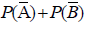Solution:
QUESTION: 24

The probability of a man hitting a target is 3/4. He tries 5 times. The probability that the target will be hit at least 3 times, is

Solution:
QUESTION: 25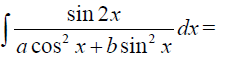Solution:
QUESTION: 26
The value of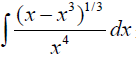Solution:
QUESTION: 27is equal to

Solution:
QUESTION: 28

If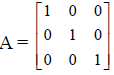, then  A2 + 2A  equals

Solution:
QUESTION: 29
The inverse of a symmetric matrix is a matrix which is
Solution:
QUESTION: 30

If a, b and c are non-zero real numbers, then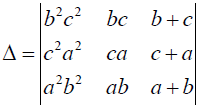is equal to

Solution: